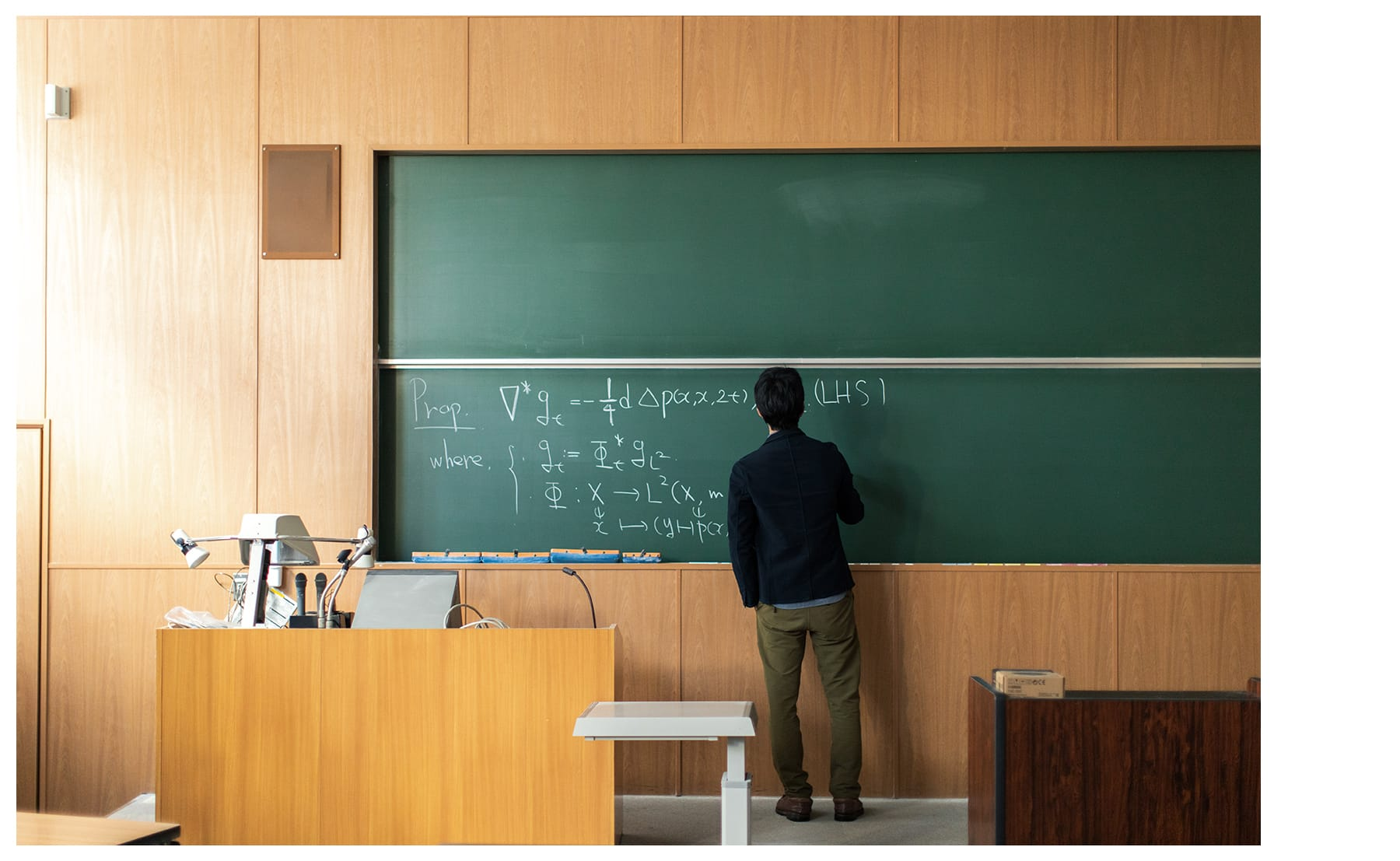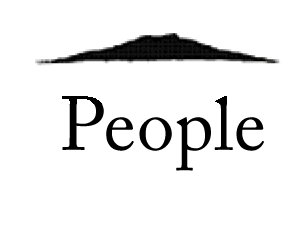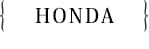#• Name：Shohei HONDA
• Position：Professor of Mathematics
• Hometown：Osaka prefecture
• Favorite Books：Recently, I've been reading Demon Slayer: Kimetsu no Yaiba, Attack on Titan, Dragon Ball Super, and Jojolion.I read only math books or comics.
• Research area：Riemannian geometry
• Posted Date：Jul 26, 2021

### １．What kind of the research are you doing?

I am studying geometric analysis on possibly singular spaces.

For example, let us consider a sphere which is regarded as the earth.

Then the sum of angles of any triangle on the sphere is not equal to pi. More precisely it is greater than pi.

This result can be understood by using ``curvature'' which is a central notion in geometry. Moreover, the difference between the sum and pi can be written by using the area of the triangle.

Next let us consider a cube which is a typical example of singular spaces. The singular points consist of vertices. Then it is natural to ask whether the sum of angles of any triangle on the cube is equal to pi or not.

I am interested in such geometric/analytic problems and studying them by using heat equations on possibly singular spaces.

### ２．What is the reason for starting your study?

When I was a master course student, I chose the book my supervisor recommended. I have been studying the same topic ever since then.

### ３．Message for prospective students

I hope everyone has a great dream you are very interested in and enjoy it.

TOPNext